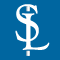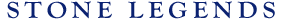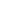HOMEPRODUCTSIDEA CENTERSERVICESTECHNICALABOUT USHello Guest Login  |  Become Member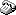800-398-1199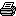214-398-1293MapEmail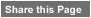Click to Share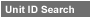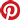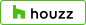Select which variables you would like to search by.Type DescriptionPrimary ViewPrimary ModifierUnit NameView Definitions

View Definitions

View DefinitionsX-Dim Y-Dim Z-Dim XX-Dim YY-Dim ZZ-Dim R-Dim D-Dim P-Dim
 Click column heading to re-sort results list.Product Name Primary View Modifier Pri Dimx  y  z Alt Dimxx  yy  zz Alt2 Dimr  d  p E\$timated Thumbnail Basil3 PoolCoping, Cast X = 4" Y = 12"Z = 4-5/8" XX = n/a YY = n/aZZ = 3-5/8" R = n/a D = n/aP = n/a \$ 7.10Basil3 PoolCoping, Cast Rectangular X = 146-7/8" Y = 146-7/8"Z = 4-5/8" XX = 87-3/8" YY = 155-1/8"ZZ = n/a R = n/a D = n/aP = n/a \$ 287.55Bullard3 PoolCoping, Cast Rectangular X = 3" Y = 12"Z = 18" XX = n/a YY = n/aZZ = n/a R = n/a D = n/aP = n/a \$ 12.96Bullnose6 PoolCoping, Cast X = 2" Y = n/aZ = 10-5/8" XX = n/a YY = n/aZZ = 8-7/8" R = n/a D = n/aP = 8-7/8" \$ 7.00BullnosePoolCoping1 PoolCoping, Cast X = 2-1/2" Y = 12"Z = 14" XX = 2" YY = n/aZZ = 13" R = n/a D = n/aP = n/a \$ 8.40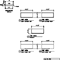BullnosePoolCoping2 PoolCoping, Cast X = 2-1/2" Y = 12"Z = 18" XX = 2" YY = n/aZZ = 17" R = n/a D = n/aP = n/a \$ 10.80BullnosePoolCoping3 PoolCoping, Cast X = 3" Y = 12"Z = 21-1/4" XX = 2-1/2" YY = n/aZZ = 20" R = n/a D = n/aP = n/a \$ 15.30Bunker1 PoolCoping, Cast X = 9" Y = 12"Z = 20-3/4" XX = n/a YY = n/aZZ = 20" R = n/a D = n/aP = 5" \$ 23.00Chamber1 PoolCoping, Cast X = 4" Y = 12"Z = 9" XX = n/a YY = n/aZZ = n/a R = n/a D = n/aP = 7-1/2" \$ 8.77Flatface PoolCoping, Cast SpecialShape X = 294" Y = 150-1/4"Z = 12" XX = n/a YY = n/aZZ = n/a R = 248-3/8" D = n/aP = n/a \$ 475.17Marsalla1 PoolCoping, Cast X = 3" Y = 12"Z = 12-1/16" XX = 1-7/8" YY = n/aZZ = 11" R = n/a D = n/aP = n/a \$ 9.76Oak1 PoolCoping, Cast X = 3" Y = 12"Z = 14-1/4" XX = n/a YY = n/aZZ = 12-1/4" R = n/a D = n/aP = n/a \$ 10.26Ogee5 PoolCoping, Cast Rectangular X = 5" Y = 12"Z = 3-3/4" XX = n/a YY = n/aZZ = 1-13/16" R = n/a D = n/aP = n/a \$ 7.00OgeeDown1 Pool PoolCoping, Cast Circular X = 3" Y = 120-1/2"Z = 120-1/2" XX = n/a YY = n/aZZ = n/a R = 60-1/4" D = 120-1/2"P = n/a \$ 410.10Record1 PoolCoping, Cast X = 5-1/2" Y = 12"Z = 3-1/2" XX = n/a YY = n/aZZ = 2" R = n/a D = n/aP = n/a \$ 7.00Wheat1 PoolCoping, Cast X = 5-1/4" Y = 12"Z = 7-1/2" XX = 3-3/4" YY = n/aZZ = n/a R = n/a D = n/aP = 1" \$ 7.04